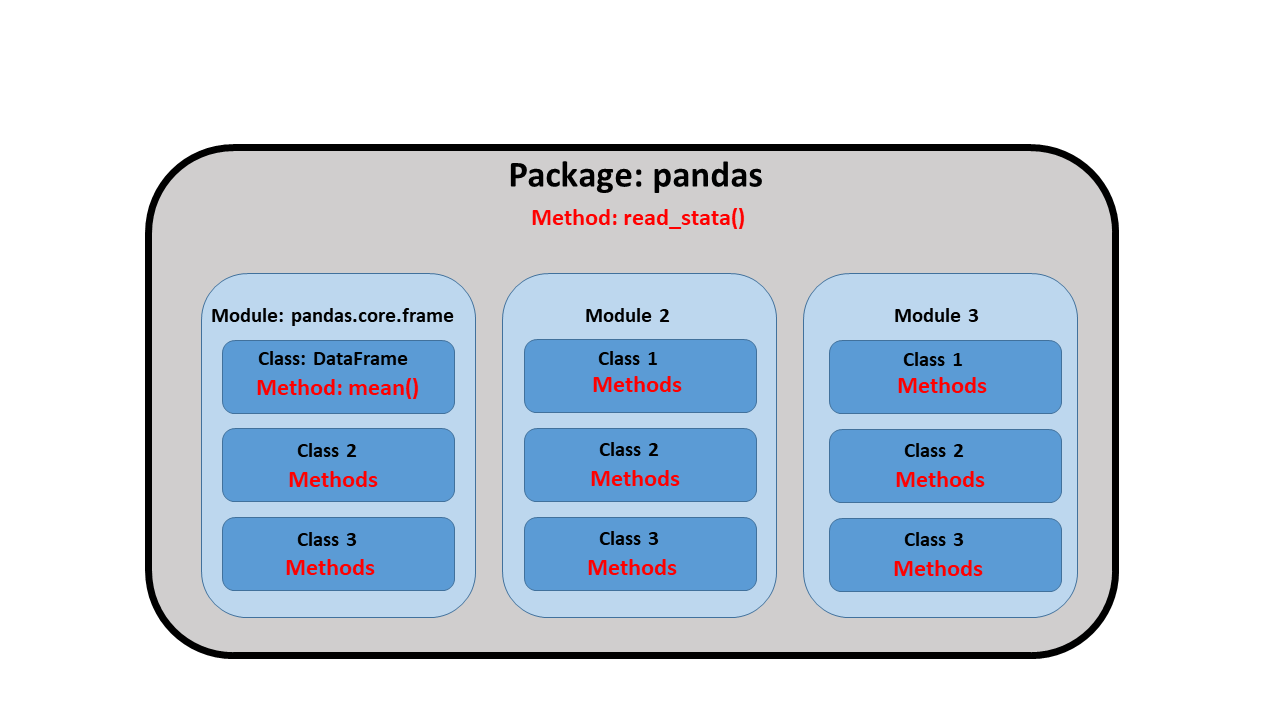Home > Programming > Stata/Python integration part 4: How to use Python packages

## Stata/Python integration part 4: How to use Python packages

In my last post, I showed you how to use pip to install four popular packages for Python. Today I want to show you the basics of how to import and use Python packages. We will learn some important Python concepts and jargon along the way. I will be using the pandas package in the examples below, but the ideas and syntax are the same for other Python packages.

Using packages with import modules

pandas is a popular Python package used for importing, exporting, and manipulating data. The package contains different modules for working with different data structures such as series, data frames, and panels.

Let’s begin by typing python which pandas to verify that pandas is installed in our system.

```. python which pandas
<module 'pandas' from 'C:\\Users\\ChuckStata\\AppData\\Local\\Programs\\Python\\
> Python38\\lib\\site-packages\\pandas\\__init__.py'>
```

If you do not see a path in your results, you will need to install the pandas package as described in my previous post.

Next, we can tell Python that we wish to use the pandas package by typing import pandas at the top of our code block. This imports the pandas module from the package.

```python
import pandas
end
```

We can then read Stata’s auto dataset from the Stata Press website into a pandas data frame named “auto” using the pandas.read_stata() method.

```python
import pandas
end
```

The term method is used to describe a function within a module. The method read_stata() is a function within the pandas package that reads Stata datasets and converts them to pandas data frames.

Import modules using an alias

The pandas module includes many methods, and we may eventually grow tired of typing pandas before each method. We can avoid typing the module name by giving it an alias. We can assign an alias to a module by typing import modulename as alias. In the code block below, I have typed import pandas as pd to assign the alias pd to pandas. Now, I can use the read_stata() method by typing pd.read_stata() rather than typing pandas.read_stata().

```python
import pandas as pd
end
```

Using methods and classes within modules

Modules are a way of subdividing the functionality of a package for different situations. A module can have a set of methods, classes, and variables defined. We can refer to them within a module in our Python statements. For example, the DataFrame class is contained in the pandas.core.frame module within the pandas package. Often, we simply refer to a class within a package and omit the name of the module. For example, the fourth line in the code block below uses the mean() method in the DataFrame class of the pandas package to estimate the mean of mpg and weight.

```python
import pandas as pd
pd.DataFrame.mean(auto[['mpg','weight']])
end
```

The code block above produces the following output.

```. python
----------------------------------------------- python (type end to exit) ------
>>> import pandas as pd
>>> pd.DataFrame.mean(auto[['mpg','weight']])
mpg         21.297297
weight    3019.459459
dtype: float64
>>> end
--------------------------------------------------------------------------------
```

Importing methods and classes from modules

You can also import classes from modules within packages and omit the module name or alias when you use the class. The third line of the code block below imports the DataFrame class from the pandas package. Note that capitalization is important. Now, you can use the mean() method by typing DataFrame.mean() rather than pd.DataFrame.mean().

```python
import pandas as pd
from pandas import DataFrame
DataFrame.mean(auto[['mpg','weight']])
end
```

Importing functions and classes using an alias

You may grow tired of typing DataFrame.mean() every time you estimate a mean. Fortunately, you can assign an alias to a class by typing from modulename import classname as alias. In the third line of the code block below, I have assigned the alias df to the DataFrame class by typing from pandas import DataFrame as df. Now, I can use the mean() method by typing df.mean() rather than DataFrame.mean().

```python
import pandas as pd
from pandas import DataFrame as df
df.mean(auto[['mpg','weight']])
end
```

Review and conclusion

Let’s review the concepts and jargon we have learned using the following diagram.A Python package is a collection of modules. Each module can contain a set of methods, classes, and variables. For example, the pandas package includes a collection of classes and methods used for importing, exporting, and managing data.

A method is a function that can accept arguments and does something. Methods can be part of a module or a class.

The methods within packages are often subdivided into modules and classes. Packages and classes can each include many methods. We must import modules or classes before we can use their methods.

You can read more about the pandas package in the pandas user guide.

Now, we have laid all the groundwork we need and are ready for the fun part! In my next post, I’ll show you how to use Stata to estimate marginal predictions from a logistic regression model and use Python to create a three-dimensional surface plot of those predictions.

Categories: Programming Tags: